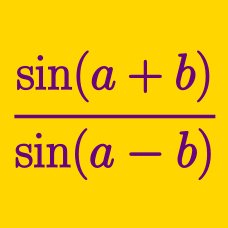Geometry

Half Angle Tangent Substitution

If $\tan \frac{x}{2}=\frac{1}{4}$, the value of $\cos x$ can be expressed as $\frac{a}{b}$, where $a$ and $b$ are coprime positive integers. What is the value of $a+b$?

If $\tan \theta = t$, which of the following is equal to $\tan 2 \theta$?

If $\tan \frac{x}{2}=-11$, the value of $\tan x$ can be expressed as $\frac{a}{b}$, where $a$ and $b$ are coprime positive integers. What is the value of $a+b$?

If $\tan \theta = t$, which of the following is equal to $\cos 2 \theta$?

If $\tan x = 2$, then $\sin 2x + \cos 2x + \tan 2x = -\frac{a}{b}$, where $a$ and $b$ are coprime positive integers. What is the value of $a+b$?

×